# eQuizShow

## Intro to Algebra 2 Final Review

### Polynomials

Question: Find the sum:

(4x2 - 5x + 3) + (7x2 +4x +7)

11x2 - x + 10

Question:               Simplify:

(17x2 + 8x - 12) - (3x2 + 4x - 8)

14x2 + 4x - 4

Question:            Simplify:

(4x + 5)(3x - 8)

12x2 - 17x - 40

Question:         FACTOR:

7x2 - 49x

7x(x - 7)

Question:                    FACTOR:

x2 + x - 56

(x + 8)(x - 7)

### Irrational Expressions

Question: Simplify: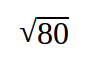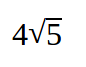Question: Simplify: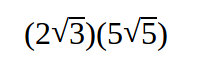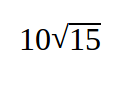Question: Simplify: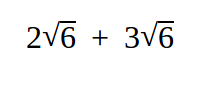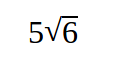Question: Simplify: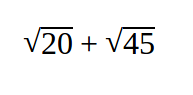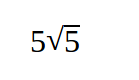Question: Rationalize the denominator: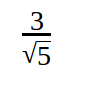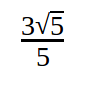### Rational Expressions

Question:
State where this expression is undefined.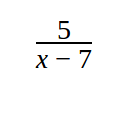x = 7

Question: Simplify: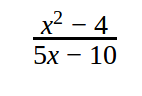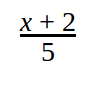Question: Simplify: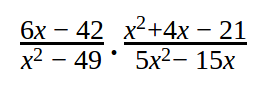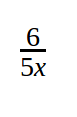Question: Combine the terms: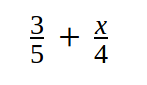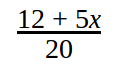Question: Simplify: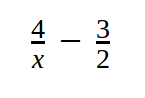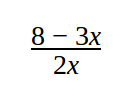### Solving Equations

Question: Solve for all values of x:

7(x + 4) + 1 = 5 - 2(x + 6)

x = - 4

Question:             Solve for x:

x2 +3x - 18 = 0

x = - 6,  x = 3

Question:          Solve for x:

| 4x - 5 | - 2 = 13

x = 5,  x = - 2.5

Question:     Solve the equation for x:

√(2x+16) = x + 4

x = 0

Question:              Solve for x:

x/3+2/5=3

x = 7.8

### Functions

Question:            Is the following a function?

(2, 3)  (4, 7)  (5, 5)  (2, 0)

NO

Question: Is the following a function?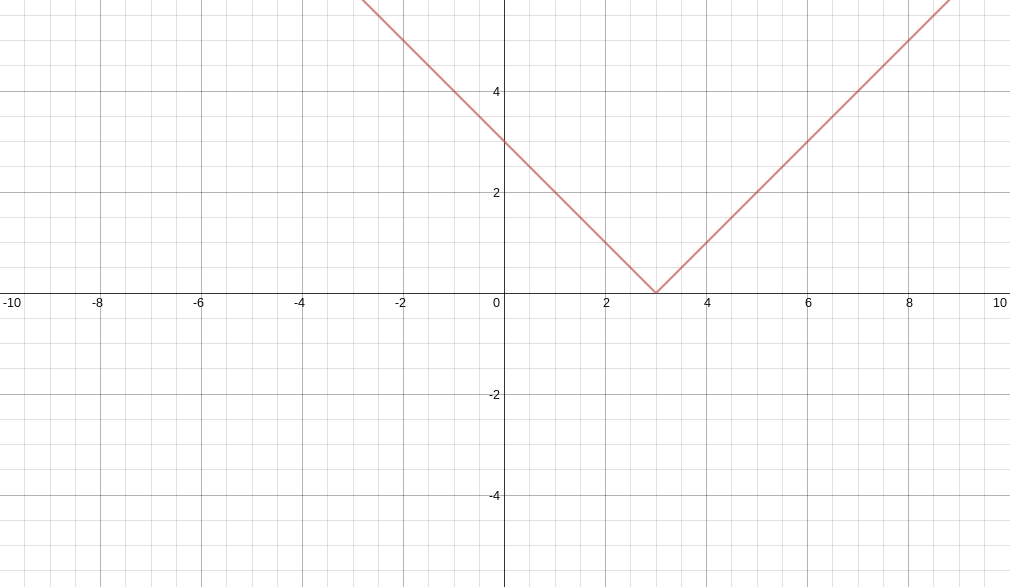YES

Question:
Find the value of f(-3) when f(x) = 7x - 2

- 23

Question:

Find the domain of  f(x) = x2 + 8x + 15